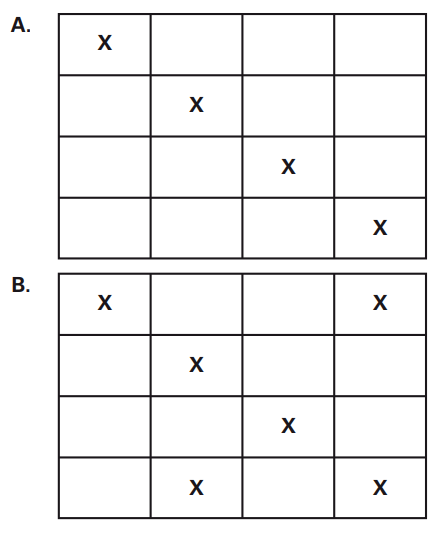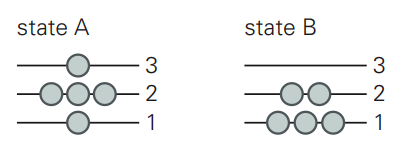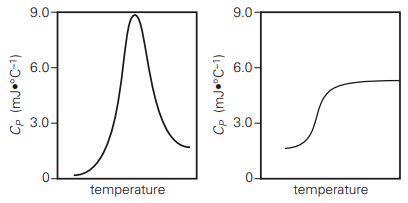# BIEN135 Final Discussion Questions (Ch. 6-8)Studied by 45 People
0.0(0)
Get a hint
hint

6.1) Which of the following properties are extensive (choose all that apply): a. temperature b. pressure c. amount of heat released d. density e. energy f. molarity g. number of moles h. mass i. volume

1/25

Tags & Description

## Studying Progress

New cards
25
Still learning
0
Almost done
0
Mastered
0
25 Terms
New cards

6.1) Which of the following properties are extensive (choose all that apply): a. temperature b. pressure c. amount of heat released d. density e. energy f. molarity g. number of moles h. mass i. volume

C, E, G, H, I

New cards
New cards

6.2) A piston containing an ideal gas expands isothermally from 7 atm pressure to 2 atm pressure. The energy of the system (that is, the contents of the piston) remains constant during this process. (True/False)

True

New cards
New cards

6.4) The enthalpy change for a process is equal to the heat transferred to the system under which of the following conditions? a. constant volume b. constant pressure c. constant temperature d. reversible expansion e. expansion at a constant pressure followed by reversible compression

B

New cards
New cards

6.8) A reaction that releases energy is called an __________ reaction.

exothermic

New cards
New cards

6.10) Electrostatic interactions are governed by _______'s Law

Coulomb

New cards
New cards

6.12) The energy for van der Waals interactions approaches _______ as the distance between atoms goes to zero and approaches _______ at infinite distance.

infinity, zero

New cards
New cards

6.17) The complete oxidation of one mole of a sugar produces carbon dioxide and water. 2000 kJ of heat is transferred from the system to the surroundings. The rearrangement of bonds as 0.5 moles of the sugar are oxidized generates heat in an open test tube (101 J*L^-1 pressure and 300 K temperature). What is the change in internal energy of the system (ΔU)? What is the change in enthalpy of the system (ΔH)?

ΔU = q + w. There is no work done. Therefore: ΔU = q = –2000 kJ•mol–1 × 0.5 mol released by the system ΔU = –1000 kJ ΔH = ΔU + PΔV. Since there is no volume change, ΔV = 0 and ΔH = ΔU = –1000 kJ.

New cards
New cards

6.19) How much kinetic energy does a system containing 3 moles of an ideal gas at 300 K possess? What is the heat capacity at constant volume? How much heat would need to be transferred to the system to raise the temperature by 15°C?

K.E. = 3/2 nRT = 3/2 × 3 mol × 8.31 J•K^(–1)•mol^(–1) × 300 K = 11,218.5 J = 11.2 kJ CV = 3/2 nR = 3/2 × 3 mol × 8.31 J•K^(–1)•mol^(–1) = 37.4 J•K^(–1) q = CV × ΔT = 37.4 J•K^(–1) × 15 K = 561 J

New cards
New cards

6.25) A salt bridge between an arginine and a glutamic acid has the two bridging atoms 3.5 Å apart. Assume that these bridging atoms have elementary charges of +1 and –1, respectively. What is the energy if the residues are a) on the protein surface (ε = 80) and b) in the core of the protein (ε = 2)?

a. U = 1/ε (qiqj/rijÅ) x 1390 kJ•mol^(–1) U = 1/80(-1/3.5) x 1390 kJ•mol^(–1)= –4.96 kJ•mol^-1 b. U = 1/ε (qiqj/rijÅ) x 1390 kJ•mol^(–1) U = 1/2(-1/3.5) x 1390 kJ•mol^(–1)= –199 kJ•mol^-1

New cards
New cards

7.1) It is easier to predict the bulk behavior of a small number of molecules than a large number of molecules. (True/False)

False

New cards
New cards

7.3) Consider a coin with two sides (H = heads; T = tails). The probability of observing HHHHHTTTTT is equal to the probability of observing HTHTHTHTHT. (True/False)

True

New cards
New cards

7.7) A state corresponds to many different microstates. (True/False)

True

New cards
New cards

7.9) When the volume of a system increases, its multiplicity ________.

increases

New cards
New cards

7.11) The combined entropy of the system and surroundings always _______________ for a spontaneous process.

increases

New cards
New cards

7.13) A coin is weighted deliberately so that the probability of tossing heads is twice the probability of tossing tails. What is the probability of tossing three heads in a row? What is the probability of tossing three tails in a row? What is the relative probability of tossing three heads versus three tails?

increases 2/3 chance of heads, 1/3 of tails. 3 heads in a row = (2/3)3 = 8/27 = 0.296. 3 tails in a row = (1/3)3 = 1/27 = 0.037. Relative probability = (8/27)/(1/27) = 8 times more likely

New cards
New cards

7.17) Consider the systems below consisting of different numbers of identical molecules (indicated by the symbol X) and equal-sized grid boxes that are either empty or occupied by a molecule. Which system has a higher entropy? What is the difference in entropy between A and B?

WA = 16!/12!4! WB = 16!/6!10! WB > WANew cards
New cards

7.20) System A has a multiplicity of 15, whereas System B has a multiplicity of 12. What is the total entropy of Systems A and B?

The entropies of the two parts will be additive. Therefore we can calculate them separately. SA = kB ln(15) = 2.71 kB; SB = kB ln(12) = 2.48 kB. Total entropy = 5.19 kB.

New cards
New cards

8.2) If the second-lowest energy level is separated from the ground state by 0.5kBT, then the second-lowest energy level will not be occupied appreciably. (True/False)

False

New cards
New cards

8.6) After spontaneous heat transfer between systems, the overall multiplicity is: a. lower than it was before the transfer of heat b. zero c. maximized d. minimized

C

New cards
New cards

8.8) The Boltzmann distribution describes the energy of molecules at _________________.

equilibrium

New cards
New cards

8.10) Kinetic energy is due to the __________ of atoms. Potential energy is due to the ___________ of atoms.

motion, configuration/relative positions

New cards
New cards

8.12) If two systems of different temperatures are brought together, there will be a net transfer of heat from the system of __________ temperature to the system of _________ temperature.

higher, lower

New cards
New cards

8.14) A system starts with a multiplicity of 1099. Heat is transferred out of the system reversibly at 298 K such that the final multiplicity is 105. How much heat was lost?

∆S = kB ln(Wfinal/Winitial) = kB ln (10^5/10^99) = -216J*K^-1 qrev = T∆S = (298 K)(–216 J•K^–1) = -64.5 kJ 64.5 kJ of heat are lost

New cards
New cards

8.17) Consider a system with five molecules and three energy levels. The energy levels are such that a molecule at energy level 1 contributes 1 J of energy to UTOTAL, a molecule at energy level 2 contributes 2 J of energy to UTOTAL, and a molecule in energy level 3 contributes 3 J of energy to UTOTAL. Which has more internal energy, state A or state B?

For State A UTOTAL = ΣNiUi = 3 × N3 + 2 × N2 + 1 × N1 = 3 × 1 + 2 × 3 + 1 × 1 = 10 For State B UTOTAL = ΣNiUi = 3 × N3 + 2 × N2 + 1 × N1 = 3 × 0 + 2 × 2 + 1 × 3 = 7 Therefore, system A has greater energy.New cards
New cards

6.20) Below are two graphs of heat capacity changes in a differential scanning calorimeter experiment. In the experiment, protein molecules in solution are unfolded by increasing the temperature. In both graphs, temperature increases from left to right. Which graph correctly characterizes the heat capacity changes of a protein as it is heated? Which characteristics in the graph lead to this conclusion?

The graph on the left is correct. Both graphs correctly indicate that the heat capacity at low temperatures, when the protein is folded, is lower than the heat capacity at high temperatures, when the protein is unfolded. However, the heat capacity of a protein peaks at its melting temperature because relatively more energy goes into breaking intramolecular interactions. It then decreases at higher temperatures when fewer unbroken interactions persist. Only the left graph indicates this correctly.New cards